Courses

# Test: Organic Chemistry

## 30 Questions MCQ Test Chemistry for JEE | Test: Organic Chemistry

Description
This mock test of Test: Organic Chemistry for Class 11 helps you for every Class 11 entrance exam. This contains 30 Multiple Choice Questions for Class 11 Test: Organic Chemistry (mcq) to study with solutions a complete question bank. The solved questions answers in this Test: Organic Chemistry quiz give you a good mix of easy questions and tough questions. Class 11 students definitely take this Test: Organic Chemistry exercise for a better result in the exam. You can find other Test: Organic Chemistry extra questions, long questions & short questions for Class 11 on EduRev as well by searching above.
QUESTION: 1

Solution:
QUESTION: 2

Solution:
QUESTION: 3

### In which of the following molecules resonance takes place through out the entire system

Solution:
QUESTION: 4

In which of the following molecules – NO2 group is not coplanar with phenyl ring ?

Solution:
QUESTION: 5

In which of the following molecels both phenyl rings are not coplanar ?

Solution:
QUESTION: 6

In which of the following molecules, all atoms are not coplanar ?

Solution:
QUESTION: 7

In which of the following, functional group isomerism is not possible?

Solution:
QUESTION: 8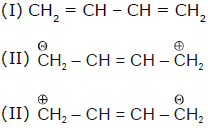Among, these, which are canonical structures ?

Solution:
QUESTION: 9

Rank the following compounds in order of decreasing acidity of the indicated hydrogen :

(i)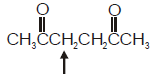(ii)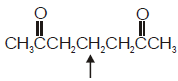(iii)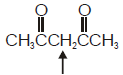Solution:
QUESTION: 10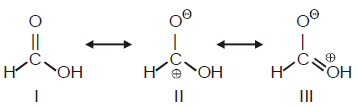Among these canonical structures, the correct order of stability is

Solution:
QUESTION: 11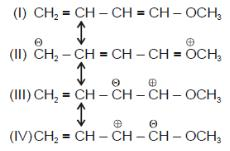Amongt these canonical structures which one is least stable ?

Solution:
QUESTION: 12

CH2 = CH – CH = CH – CH3 is more stable than CH3 – CH = C = CH – CH3 because

Solution:
QUESTION: 13

For phenol which ofthe following resonating structure is the most stable ?

Solution:
QUESTION: 14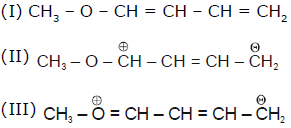Among these three canonical structures (through more are possible) what would be their relative contribution in the hybrid

Solution:
QUESTION: 15

The most stable resonating structure of following compound is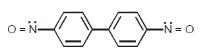Solution:
QUESTION: 16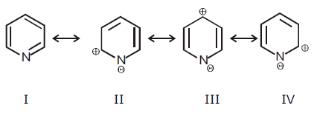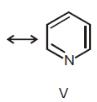Among these canonical structures of pyridiine, the correct order of stability is

Solution:
QUESTION: 17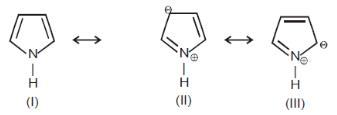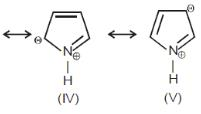Solution:
QUESTION: 18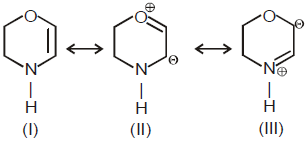The least stable canonical structure among these is

Solution:
QUESTION: 19

Rank the following compounds in order of decreasing acidity.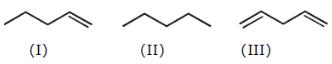Solution:
QUESTION: 20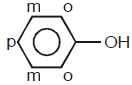In phenol, π-electron-density is maximum on

Solution:
QUESTION: 21

Which of the following compounds has maximum electron density in ring ?

Solution:
QUESTION: 22

In which of the following molecules π-electron density in ring is minimum?

Solution:
QUESTION: 23

In which of the following molecules π-electron density in ring is maximum?

Solution:
QUESTION: 24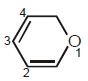In this molecules, π-electron-density is more on

Solution:
QUESTION: 25

In which of the following pairs, first species is more stable than second ?

Solution:
QUESTION: 26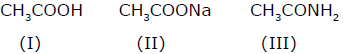Among these compounds, the correct order of resonance energy is

Solution:
QUESTION: 27

Rank the following free radicals in order of decreasing stability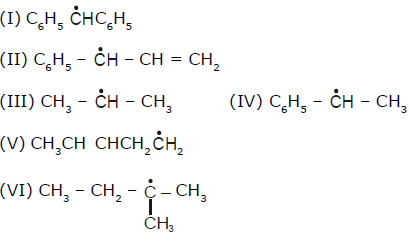Solution:
QUESTION: 28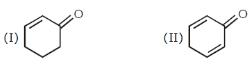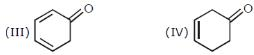Among these compounds, which one has maximum resonance energy ?

Solution:
QUESTION: 29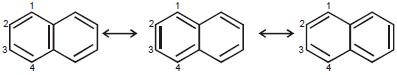There are three canonical structures of napthalene. Examine them and find correct statement among the following:

Solution:
QUESTION: 30

Which of the following has longest C – O bond:

Solution: